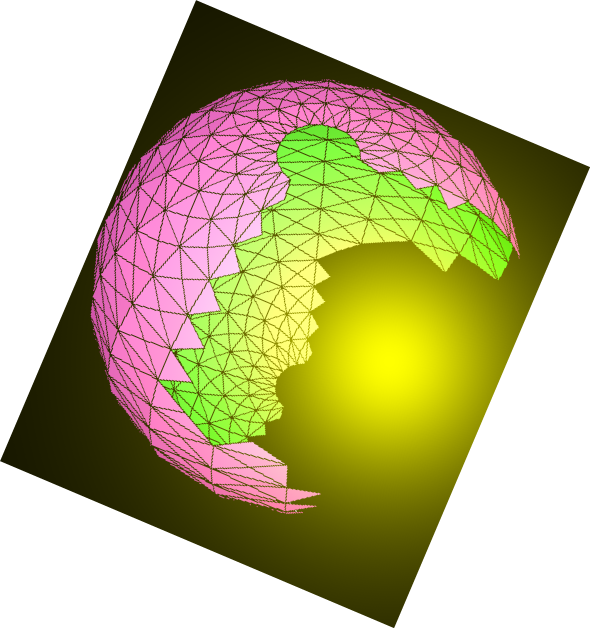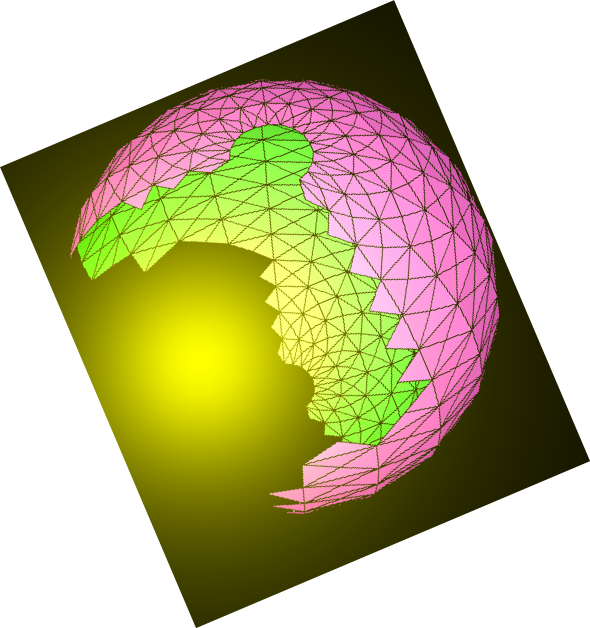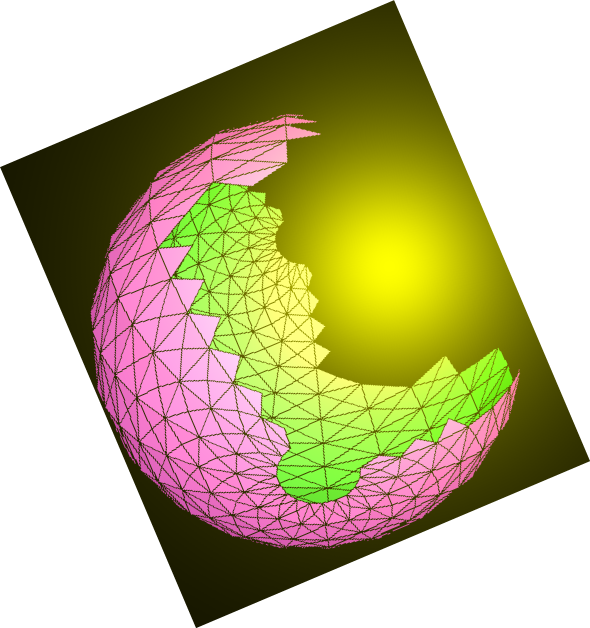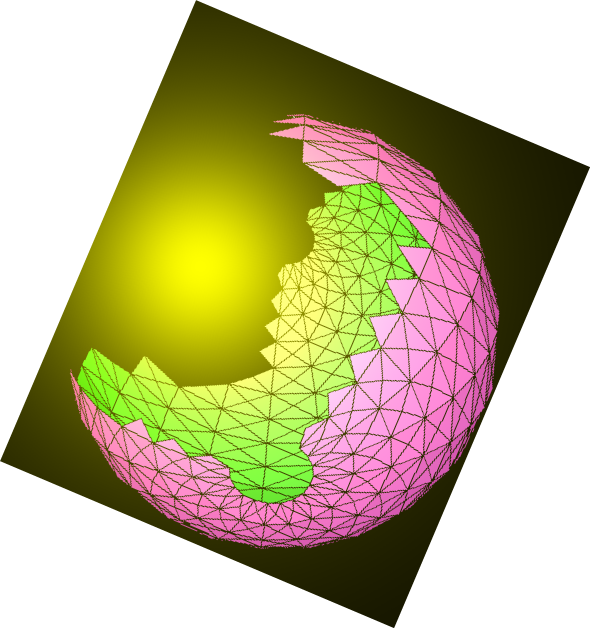# N.S.B. Cosmic Center### Elements of Fundamental Theory by Nazir S. Baaklini

Back to Books

This is a list of very concise and elegant monographs that are intended for mature graduate students and researchers who seek an overview of the corresponding fundamental topics. The author himself returns to these documents whenever he needs a refreshing session on these basic subjects.

These documents, taking the form of pdf files, may be downloaded from another web location that belongs to the author. You can obtain the needed links, and additional information, from the author at nazirbaaklini@yahoo.com.

### Elements of Fundamental Theory 1. Mechanical Principles

This account gives the fundamental principles of classical mechanics. The included topics are: Lagrangian formalism, Hamiltonian formalism, canonical transformations, Hamilton-Jacobi equation, Poisson brackets, infinitesimal transformations, constrained systems, and Fermi-Bose systems.

### Elements of Fundamental Theory 2. Electromagnetic Theory

This account gives the essentials of electromagnetic theory.The included topics are: Electrostatics, electrostatic force, electric field, electric potential, equations of electrostatics, electrostatics energy; Magnetostatics, electric currents, magnetic forces and fields, magnetic potential, magnetic flux, equations of magnetostatics, magnetostatic energy; Electrodynamics, magnetic induction, Maxwell's equations, electromagnetic waves, electromagnetic energy, and the dynamics of a charged particle.

### Elements of Fundamental Theory 3. Relativistic Framework

This account introduces Einstein's relativistic mechanics and the associated concept of four-dimensional spacetime. Subsequently Maxwell's electrodynamics is presented in this relativistic framework.

### Elements of Fundamental Theory 4. Statistical Thermodynamics

This account introduces the subjects of classical and quantum statistical thermodynamics. Topics include: Thermodynamic principles, thermodynamic functions, classical and quantum statistics, the statistical thermodynamics of ideal gases, Bose and Fermi gases, the statistics of harmonic osccilator systems, Bose-Einstein condensation, entropy and information, and the statistical thermodynamics of relativistic gas.

### Elements of Fundamental Theory 5. Quantum Theory

This account presents the basic concepts and formalism of quantum mechanics. Topics include: Quantum rules and the wave function, Planck quanta and de Broglie waves, expectation values, the hydrogen spectrum, second quantization, the Dirac equation, electromagnetic coupling, magnetic moment, and current conservation.

### Elements of Fundamental Theory 6. Lie Algebraic Tools

This account presents a compact account of the algebraic tools of matrices, tensors, and spinors, as well as, the theory of Lie algebras. Topics include: Matrix algebra, tensors, spinors, Lie algebras, orthogonal algebras, unitary algebras, symplectic algebras, and exceptional algebras.

### Elements of Fundamental Theory 7. Relativistic Fields

This account presents the basic representations of the spacetime algebra and the theory of lower spin fields. Topics include: Lorentz-Poincare transformations, Poincare algebra, representations of the Lorentz algebra, Clifford and Dirac algebras, Lorentz spinors, representations of the Poincare algebra, relativistic fields, the conformal algebra, action principles, scalar bosons, spin-1/2 fermions, vector bosons, spin-3/2 fermions, tensor bosons, and reflective symmetries.

### Elements of Fundamental Theory 8. Algebraic Gauge Principles

This account presents the basic elements of non-Abelian gauge theory and details the construction of associated Lie-algebraic systems and symmetry breaking scenarios. Topics include: Gauge algebraic formalism, symmetry breaking, orthogonal gauge system, unitary gauge system, symplectic gauge system, and exceptional gauge system.

### Elements of Fundamental Theory 9. Electronuclear Unification

We review the phenomenolgy of elementary particles and their electromagnetic, weak, and strong interactions. We present the formalism of the standard model of electroweak and strong interactions, as well as possible extensions to grand unified theories. Topics include: Subnuclear phenomenology, nuclear isospin, nucleons and pions, hadronic spectrum, neutrino and Fermi coupling; Particles and processes, hadronic SU(3), beyond SU(3), selection rules, leptons, quarks, universal (V-A) weak interaction, intermediate vector bosons; Electronuclear gauge theory, quantum chromodynamics, electroweak currents, electroweak Lagrangian, symmetry breaking and masses, effective phenomenology; Quark-lepton unification, SU(5) grand unification, symmetry breaking and baryon number, low-energy phenomenology; Alternative grand unifcation, generalized unitary scheme, classical alternatives, supersymmetric exceptional unification.

### Elements of Fundamental Theory 10. Supersymmetric Field Theory

We present a concise review of superalgebras and of supersymmetric field theory in 4-spacetime. Extended and higher-dimensional supersymmetries are also treated. Topics include: Superalgebras, supergroups, simple, unitary, orthosymplectic and exceptional superalgebras; Spacetime supersymmetry, Poincare superalgebra, supermultiplets and superfields, superfield calculus, chiral superfields, scalar and other supermultiplets; Supersymmetric gauge theory, superfield gauge formalism, symmetry breaking; Extended supersymmetries, extended Poincare superalgebra, 2-fold, 4-fold and 8-fold extended supersymmetries, six, ten and 11-dimensional supersymmetries, conformal superalgebra.

### Elements of Fundamental Theory 11. Gravidynamic Field Theory

This account presents a concise account of Einstein's gravidynamic field theory, as well as its extensions to an Einstein-Dirac, and to a supersymmetric system. Higher-dimensional extensions are also described. Topics include: Einstein principles, general covariance, covariant dynamics of scalar matter, covariant dynamics of vector matter, metric field dynamics, Einstein's equations; Vierbein theory, Einstein dynamics for the Dirac field, local Lorentz invariance, Poincare gauge strucrure, topological gravidynamics; Supergravidynamics, simple supergravidynamics, topological supergravidynamics, extended supergravidynamics, auxilliary fields and superspace; Gravidynamic theory in higher dimensions, 5-dimensional relativity, non-Abelian gauge fields, fermions in higher dimensions, 11-dimensional supergravidynamics, compactification.

Back to BooksHome
N.S.B. Cosmic Center by Nazir S. Baaklini